## Access Answers to NCERT Class 7 Maths Chapter 14 – Symmetry Exercise 14.1

### Access Answers to NCERT Class 7 Maths Chapter 14 – Symmetry Exercise 14.1

1. Copy the figures with punched holes and find the axes of symmetry for the following:

(a)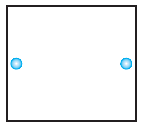Solution:-

A figure has a line of symmetry, if there is a line about which the figure may be folded so that the two parts of the figure will coincide.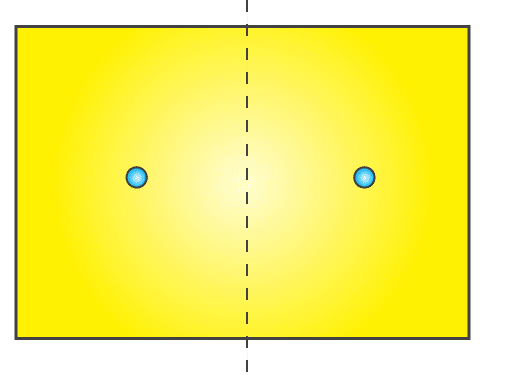(b)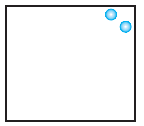Solution:-

A figure has a line of symmetry, if there is a line about which the figure may be folded so that the two parts of the figure will coincide.(c)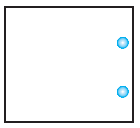Solution:-

A figure has a line of symmetry, if there is a line about which the figure may be folded so that the two parts of the figure will coincide.(d)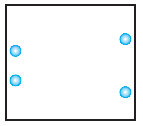Solution:-

A figure has a line of symmetry, if there is a line about which the figure may be folded so that the two parts of the figure will coincide.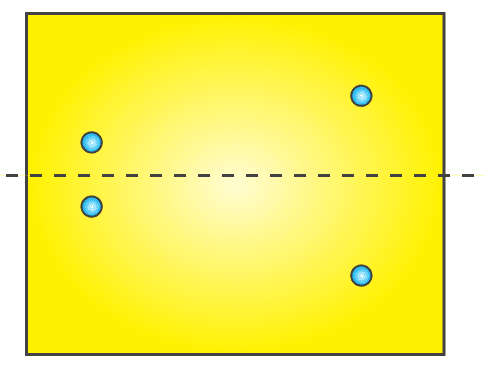(e)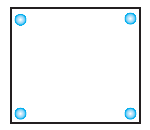Solution:-

A figure has a line of symmetry, if there is a line about which the figure may be folded so that the two parts of the figure will coincide.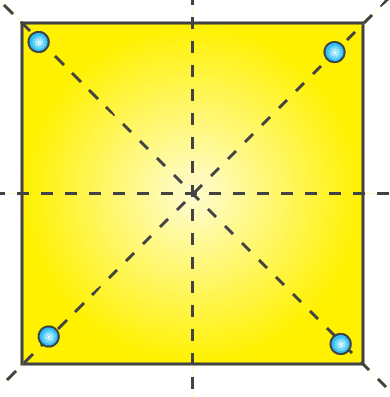(f)Solution:-

A figure has a line of symmetry, if there is a line about which the figure may be folded so that the two parts of the figure will coincide.(g)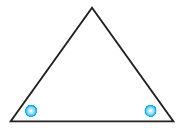Solution:-

A figure has a line of symmetry, if there is a line about which the figure may be folded so that the two parts of the figure will coincide.(h)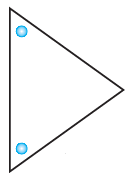Solution:-

A figure has a line of symmetry, if there is a line about which the figure may be folded so that the two parts of the figure will coincide.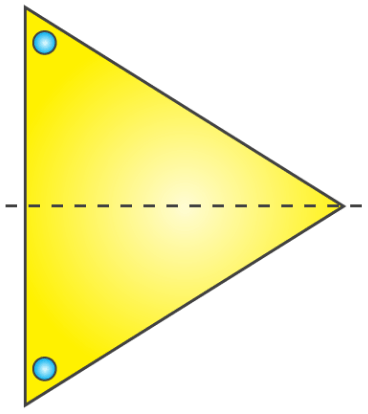(i)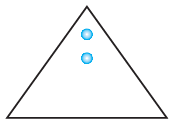Solution:-

A figure has a line of symmetry, if there is a line about which the figure may be folded so that the two parts of the figure will coincide.(j)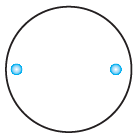Solution:-

A figure has a line of symmetry, if there is a line about which the figure may be folded so that the two parts of the figure will coincide.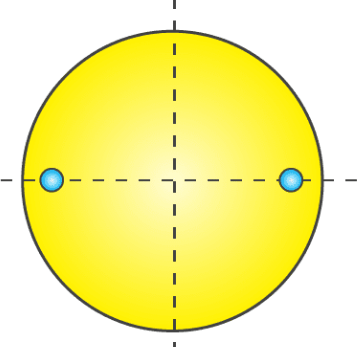(k)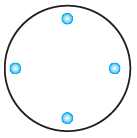Solution:-

A figure has a line of symmetry, if there is a line about which the figure may be folded so that the two parts of the figure will coincide.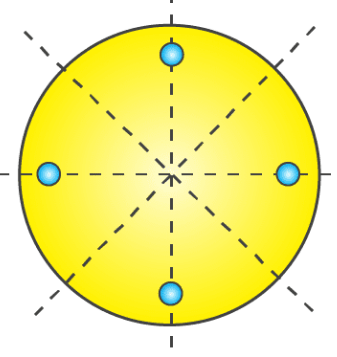(l)Solution:-

A figure has a line of symmetry, if there is a line about which the figure may be folded so that the two parts of the figure will coincide.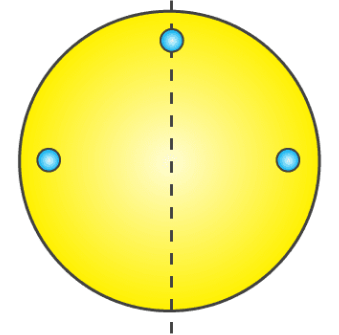2. Given the line(s) of symmetry, find the other hole(s):

(a)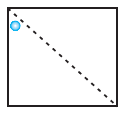Solution:-

A figure has a line of symmetry, if there is a line about which the figure may be folded so that the two parts of the figure will coincide.

So, other hole is shown in the figure below.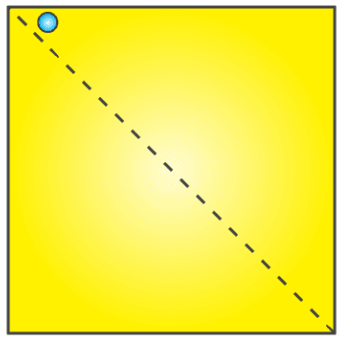(b)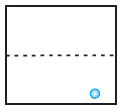Solution:-

A figure has a line of symmetry, if there is a line about which the figure may be folded so that the two parts of the figure will coincide.

So, other hole is shown in the figure below.(c)Solution:-

A figure has a line of symmetry, if there is a line about which the figure may be folded so that the two parts of the figure will coincide.

So, other hole is shown in the figure below.(d)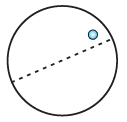Solution:-

A figure has a line of symmetry, if there is a line about which the figure may be folded so that the two parts of the figure will coincide.

So, other hole is shown in the figure below.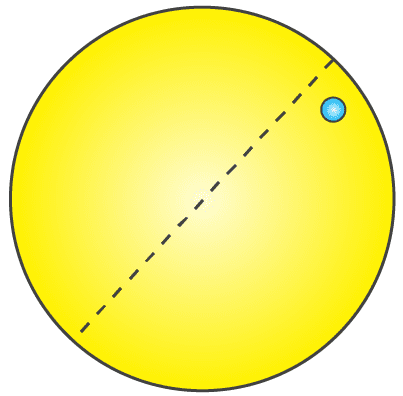(e)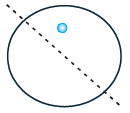Solution:-

A figure has a line of symmetry, if there is a line about which the figure may be folded so that the two parts of the figure will coincide.

So, other hole is shown in the figure below.3. In the following figures, the mirror line (i.e., the line of symmetry) is given as a dotted line. Complete each figure performing reflection in the dotted (mirror) line. (You might perhaps place a mirror along the dotted line and look into the mirror for the image). Are you able to recall the name of the figure you complete?

(a)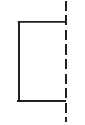Solution:-

The concept of line of symmetry is closely related to mirror reflection. A shape has line symmetry when one half of it is the mirror image of the other half. A mirror line, thus helps to visualise a line of symmetry.

While dealing with mirror reflection, care is needed to note down the left-right changes in the orientation.Name of the figure is square.

(b)Solution:-

The concept of line of symmetry is closely related to mirror reflection. A shape has line symmetry when one half of it is the mirror image of the other half. A mirror line, thus helps to visualise a line of symmetry.

While dealing with mirror reflection, care is needed to note down the left-right changes in the orientation.Name of the figure is triangle.

(c)Solution:-

The concept of line of symmetry is closely related to mirror reflection. A shape has line symmetry when one half of it is the mirror image of the other half. A mirror line, thus helps to visualise a line of symmetry.

While dealing with mirror reflection, care is needed to note down the left-right changes in the orientation.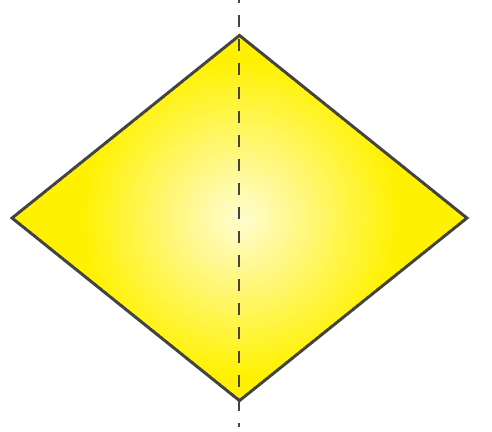Name of the figure is rhombus.

(d)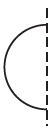Solution:-

The concept of line of symmetry is closely related to mirror reflection. A shape has line symmetry when one half of it is the mirror image of the other half. A mirror line, thus helps to visualise a line of symmetry.

While dealing with mirror reflection, care is needed to note down the left-right changes in the orientation.Name of the figure is circle.

(e)Solution:-

The concept of line of symmetry is closely related to mirror reflection. A shape has line symmetry when one half of it is the mirror image of the other half. A mirror line, thus helps to visualise a line of symmetry.

While dealing with mirror reflection, care is needed to note down the left-right changes in the orientation.Name of the figure is pentagon.

(f)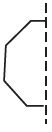Solution:-

The concept of line of symmetry is closely related to mirror reflection. A shape has line symmetry when one half of it is the mirror image of the other half. A mirror line, thus helps to visualise a line of symmetry.

While dealing with mirror reflection, care is needed to note down the left-right changes in the orientation.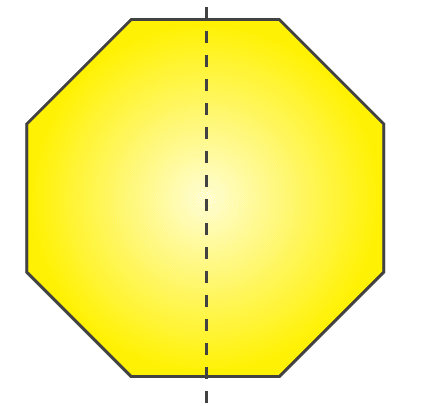Name of the figure is octagon.

4. The following figures have more than one line of symmetry. Such figures are said to have multiple lines of symmetry.Identify multiple lines of symmetry, if any, in each of the following figures:

(a)Solution:-

Figure given has 3 lines of symmetry.

So, it has multiple lines of symmetry.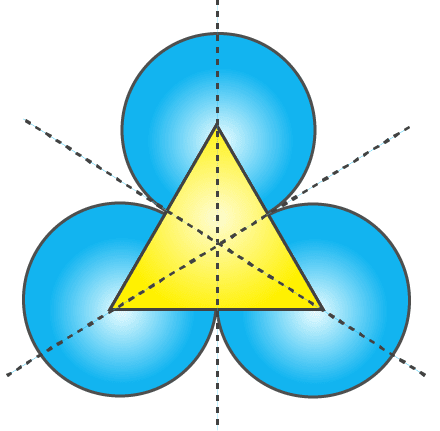(b)Solution:-

Figure given has 2 lines of symmetry.

So, it has multiple lines of symmetry.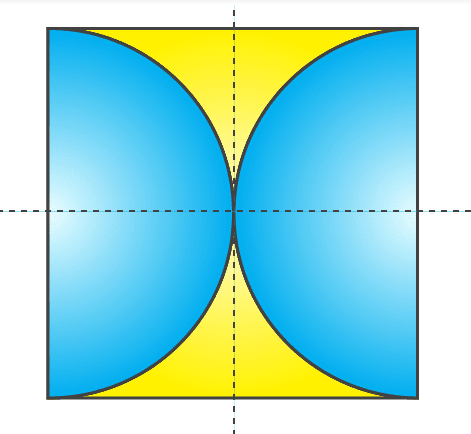(c)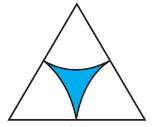Solution:-

Figure given has 3 lines of symmetry.

So, it has multiple lines of symmetry.(d)Solution:-

Figure given has 2 lines of symmetry.

So, it has multiple lines of symmetry.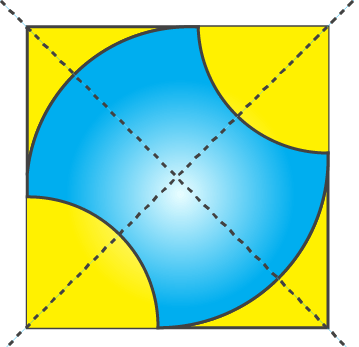(e)Solution:-

Figure given has 4 lines of symmetry.

So, it has multiple lines of symmetry.(f)Solution:-

Figure given has only 1 line of symmetry.(g)Solution:-

Figure given has 4 lines of symmetry.

So, it has multiple lines of symmetry.(h)Solution:-

Figure given has 6 lines of symmetry.

So, it has multiple lines of symmetry.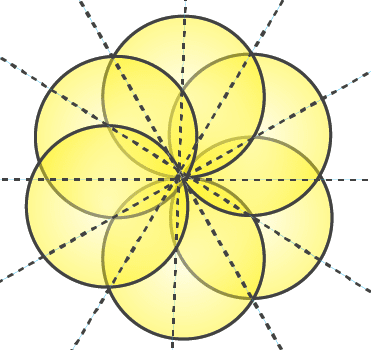5. Copy the figure given here.

Take any one diagonal as a line of symmetry and shade a few more squares to make the figure symmetric about a diagonal. Is there more than one way to do that? Will the figure be symmetric about both the diagonals?Solution:-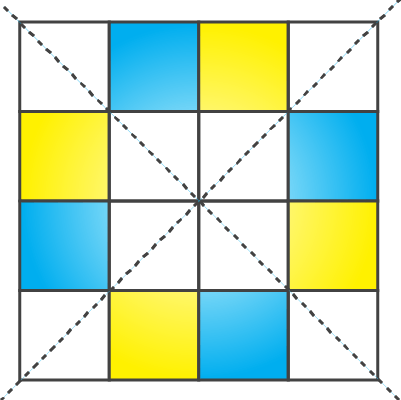By observing the above figure,

Yes, the figure will be symmetrical about both diagonals.By observing the above figure,

Yes, the figure can be made symmetrical by more than one way.

6. Copy the diagram and complete each shape to be symmetric about the mirror line(s):

(a)Solution:-

The concept of line of symmetry is closely related to mirror reflection. A shape has line symmetry when one half of it is the mirror image of the other half. A mirror line, thus helps to visualise a line of symmetry.

While dealing with mirror reflection, care is needed to note down the left-right changes in the orientation.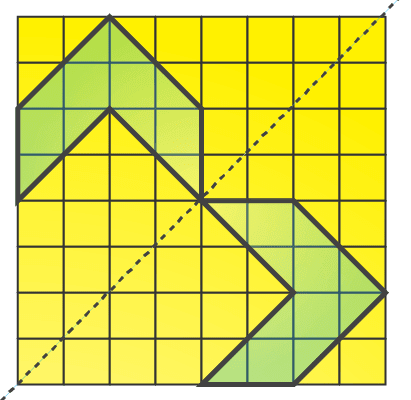(b)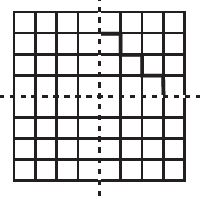Solution:-

The concept of line of symmetry is closely related to mirror reflection. A shape has line symmetry when one half of it is the mirror image of the other half. A mirror line, thus helps to visualise a line of symmetry.

While dealing with mirror reflection, care is needed to note down the left-right changes in the orientation.(c)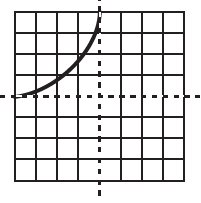Solution:-

The concept of line of symmetry is closely related to mirror reflection. A shape has line symmetry when one half of it is the mirror image of the other half. A mirror line, thus helps to visualise a line of symmetry.

While dealing with mirror reflection, care is needed to note down the left-right changes in the orientation.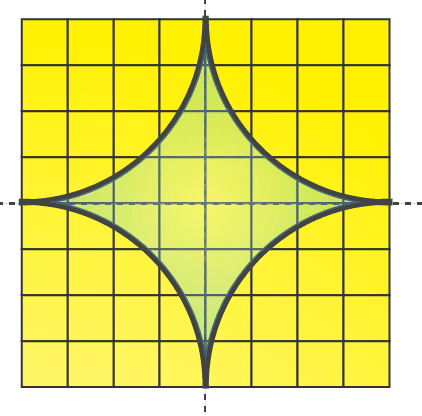7. State the number of lines of symmetry for the following figures:

(a) An equilateral triangle

Solution:-

A figure has a line of symmetry, if there is a line about which the figure may be folded so that the two parts of the figure will coincide.

An equilateral triangle has 3 lines of symmetry is shown in the figure below,(b) An isosceles triangle

Solution:-

A figure has a line of symmetry, if there is a line about which the figure may be folded so that the two parts of the figure will coincide.

An isosceles triangle has 1 lines of symmetry is shown in the figure below,(c) A scalene triangle

Solution:-

A figure has a line of symmetry, if there is a line about which the figure may be folded so that the two parts of the figure will coincide.

A scalene triangle has no line of symmetry is shown in the figure below,(d) A square

Solution:-

A figure has a line of symmetry, if there is a line about which the figure may be folded so that the two parts of the figure will coincide.

A square has 4 lines of symmetry is shown in the figure below,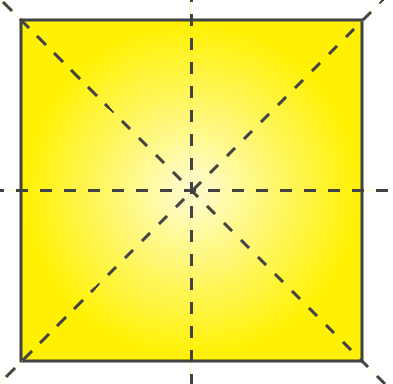(e) A rectangle

Solution:-

A figure has a line of symmetry, if there is a line about which the figure may be folded so that the two parts of the figure will coincide.

A rectangle has 2 lines of symmetry is shown in the figure below,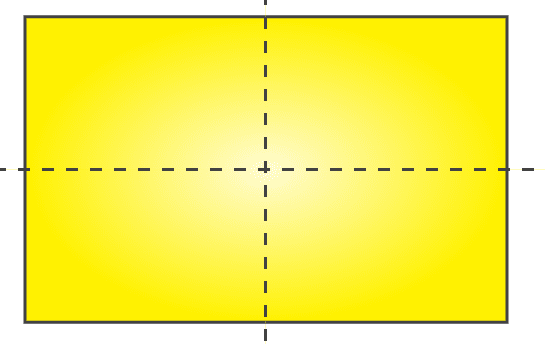(f) A rhombus

Solution:-

A figure has a line of symmetry, if there is a line about which the figure may be folded so that the two parts of the figure will coincide.

A rhombus has 2 lines of symmetry is shown in the figure below,(g) A parallelogram

Solution:-

A figure has a line of symmetry, if there is a line about which the figure may be folded so that the two parts of the figure will coincide.

A parallelogram has no line of symmetry is shown in the figure below,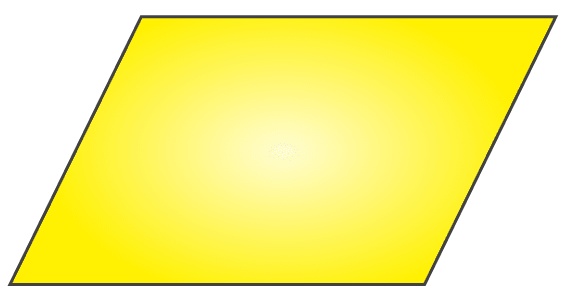Solution:-

A figure has a line of symmetry, if there is a line about which the figure may be folded so that the two parts of the figure will coincide.

A quadrilateral has no line of symmetry is shown in the figure below,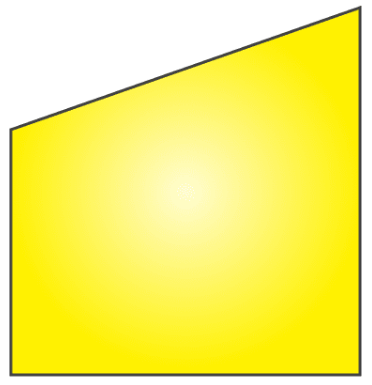(i) A regular hexagon

Solution:-

A figure has a line of symmetry, if there is a line about which the figure may be folded so that the two parts of the figure will coincide.

A regular hexagon has 6 lines of symmetry is shown in the figure below,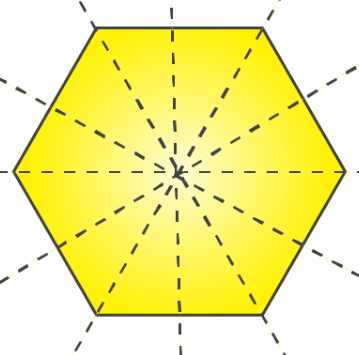(j) A circle

Solution:-

A figure has a line of symmetry, if there is a line about which the figure may be folded so that the two parts of the figure will coincide.

A circle has infinite lines of symmetry,8. What letters of the English alphabet have reflectional symmetry (i.e., symmetry related to mirror reflection) about.

(a) a vertical mirror (b) a horizontal mirror

(c) both horizontal and vertical mirrors

Solution:-

(a) a vertical mirror

The English alphabet have reflection symmetry about a vertical mirror are, A, H, I, M, O, T, U, V, W, X, Y(b) a horizontal mirror

The English alphabet have reflection symmetry about a horizontal mirror are, B, C, D, E, H, I, K, O, X(c) both horizontal and vertical mirrors

The English alphabet have reflection symmetry about both horizontal and vertical mirrors are, H, I, O, X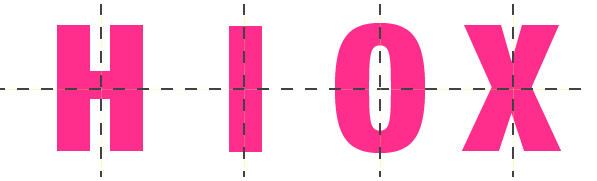9. Give three examples of shapes with no line of symmetry.

Solution:-

A shape has a no line of symmetry, if there is no line about which the figure may be folded and also parts of the figure will not coincide.

A scalene triangle, a quadrilateral and a parallelogram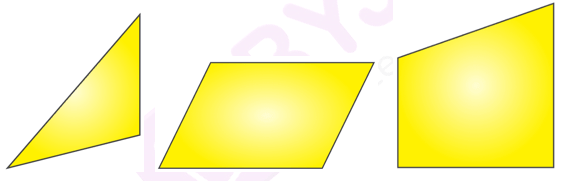10. What other name can you give to the line of symmetry of

(a) an isosceles triangle?

Solution:-

The other name to the line of symmetry of an isosceles triangle is median or altitude.

(b) a circle?

The other name to the line of symmetry of a circle is diameter.